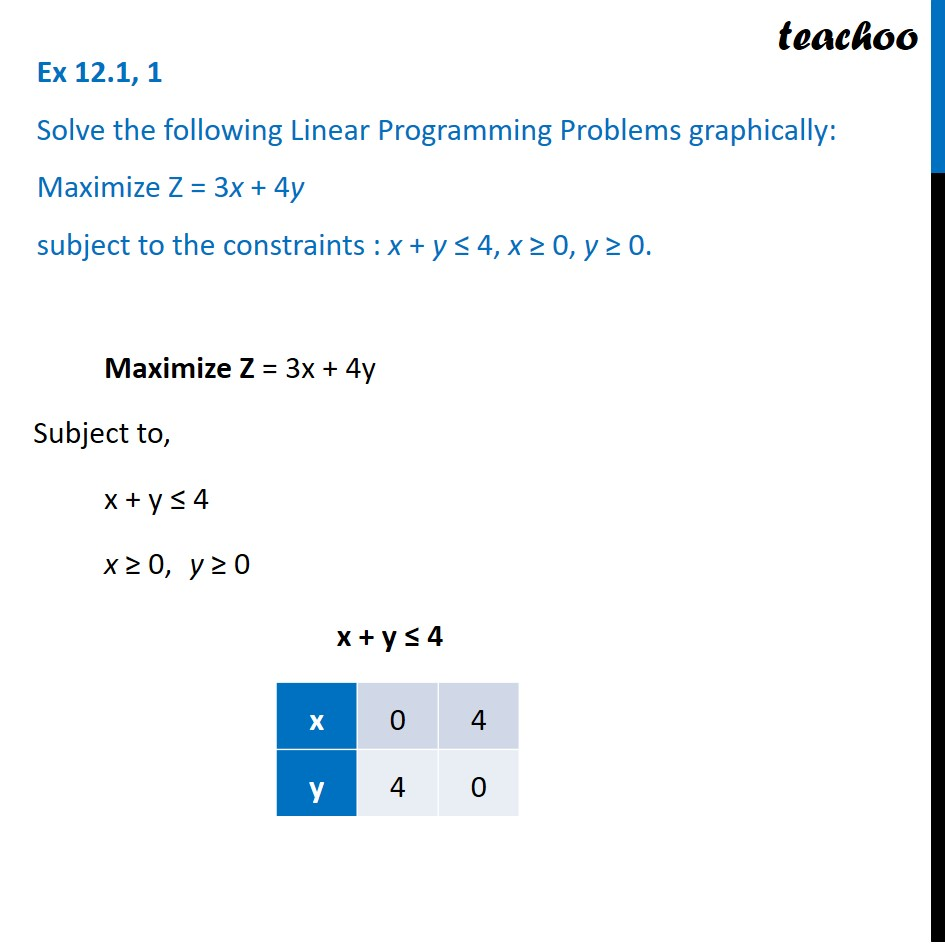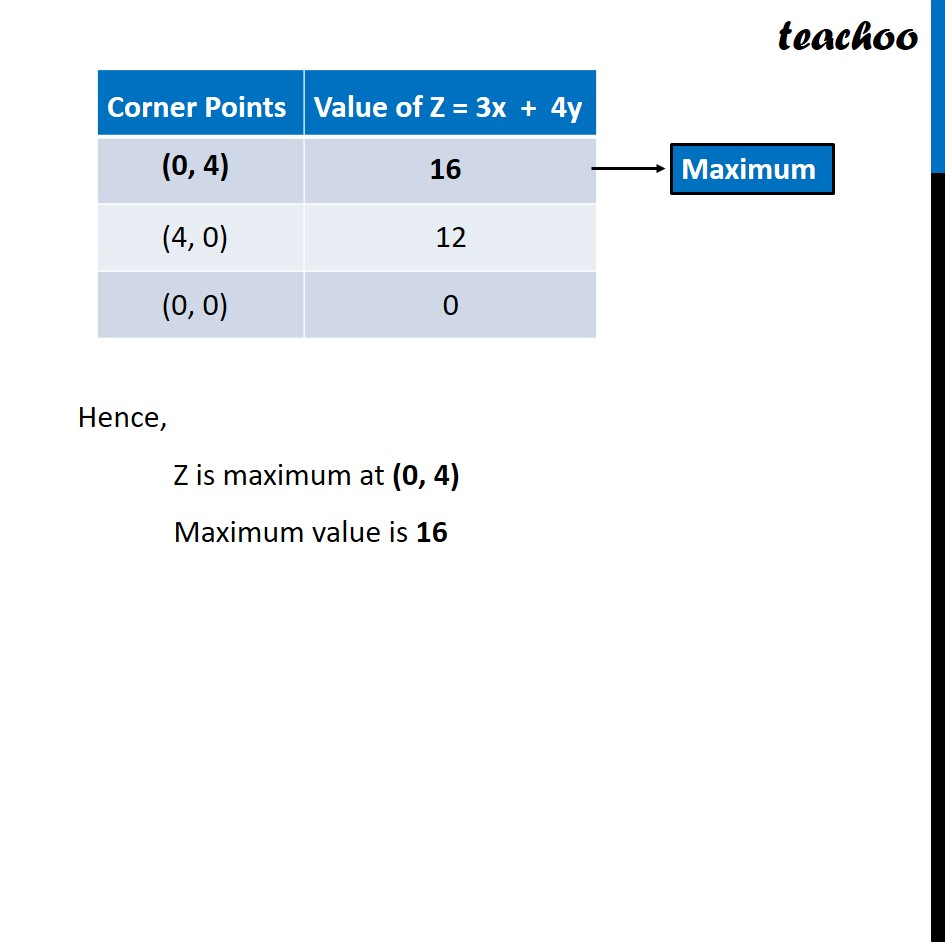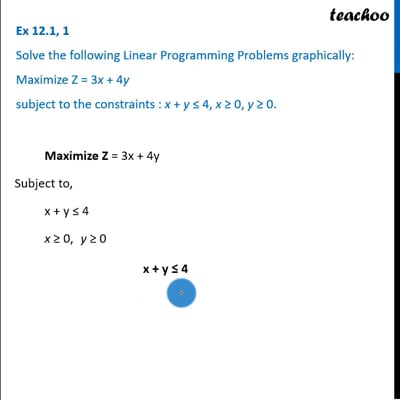Linear equations given - Bounded

Chapter 12 Class 12 Linear Programming
Concept wiseThis video is only available for Teachoo black users

Introducing your new favourite teacher - Teachoo Black, at only ₹83 per month

### Transcript

Ex 12.1, 1 Solve the following Linear Programming Problems graphically: Maximize Z = 3x + 4y subject to the constraints : x + y ≤ 4, x ≥ 0, y ≥ 0. Maximize Z = 3x + 4y Subject to, x + y ≤ 4 x ≥ 0, y ≥ 0 x + y ≤ 4 Hence, Z is maximum at (0, 4) Maximum value is 16